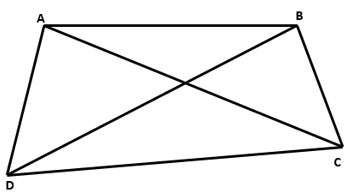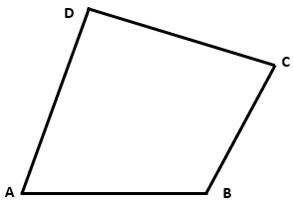## LetsPlayMaths.Com

WELCOME TO THE WORLD OF MATHEMATICS

If A, B, C, D be four points in a plane such that no three points are collinear and the line segments AB, BC, CD and DA do not intersect except their end points, then the figure formed by these four line segments is called the quadrilateral ABCD.1. The four points A, B, C, D are known as vertices
2. The four line segments AB, BC, CD, DA are known as sides
3. ∠ABC, ∠BCD, ∠CDA, and ∠DAB are called its angles
4. The line segments AC and BD are called its diagonals

The sum of all the angles of a quadrilateral is equal to 360°.∠A + ∠B + ∠C + ∠D = 360°

Example 1. Three angles of quadrilateral ABCD are ∠A = 75°, ∠B = 50°, and ∠C = 85°. Find ∠D.

Solution. We know that the sum of the angles of a quadrilateral is 360o.

∠A + ∠B + ∠C + ∠D = 360°

=> 75° + 50° + 85° + ∠D = 360°

=> 210° + ∠D = 360°

=> ∠D = 360° − 210°

=> ∠D = 150°

Hence, ∠D = 150°

Example 2. The angles of a quadrilateral are in the ratio 3 : 5 : 6 : 4. Find the measure of each angle of the quadrilateral.

Solution. Let's assume the measures of the angles of the given quadrilateral be 3p, 5p, 6p and 4p respectively.

As we know sum of angles of a quadrilateral is 360°.

3p + 5p + 6p + 4p = 360

=> 18p = 360

=> p = 20

First angle = 3p = 3 × 20 = 60°

Second angle = 5p = 5 × 20 =100°

Third angle = 6p = 6 × 20 = 120°

Fourth angle = 4p = 4 × 20 = 80°

Example 3. Three angles of a quadrilateral are in the ratio 2 : 5 : 7. If the fourth angle measures 80°, then find the other three angles of the quadrilateral.

Solution. Let's assume first angle is 2q, second angle is 5q and third angle is 7q.

As we know sum of angles of a quadrilateral is 360°.

2q + 5q + 7q + 80° = 360°

=> 14q + 80° = 360°

=> 14q = 360° − 80°

=> 14q = 280°

=> q = 20°

First angle = 2p = 2 × 20 = 40°

Second angle = 5p = 5 × 20 =100°

Third angle = 7p = 7 × 20 = 140°

Example 4. Two angles of a quadrilateral are 110° and 80° and the remaining two angles are equal. What is the measure of each of the equal angles?

Solution. Sum of given two angles = 110° + 80° = 190°

Sum of remaining two angles = 360° − 190° = 170°

We know both the remaining angles of the quadrilateral are equal.

So, each angles measures = 170°2

= 85°

So, both equal angles measure is equal to 85°.

Example 5. Three angles of a quadrilateral are in the ratio 4 : 6 : 2. If the fourth angle is 120o, find the other three angles.

Solution. Let the three angles be 4a, 6a and 2a.

4a + 6a + 2a + 120° = 360°

=> 12a = 360° − 120°

=> 12a = 240°

=> a = 20°

So, the other three angles are 80°, 120° and 40°.